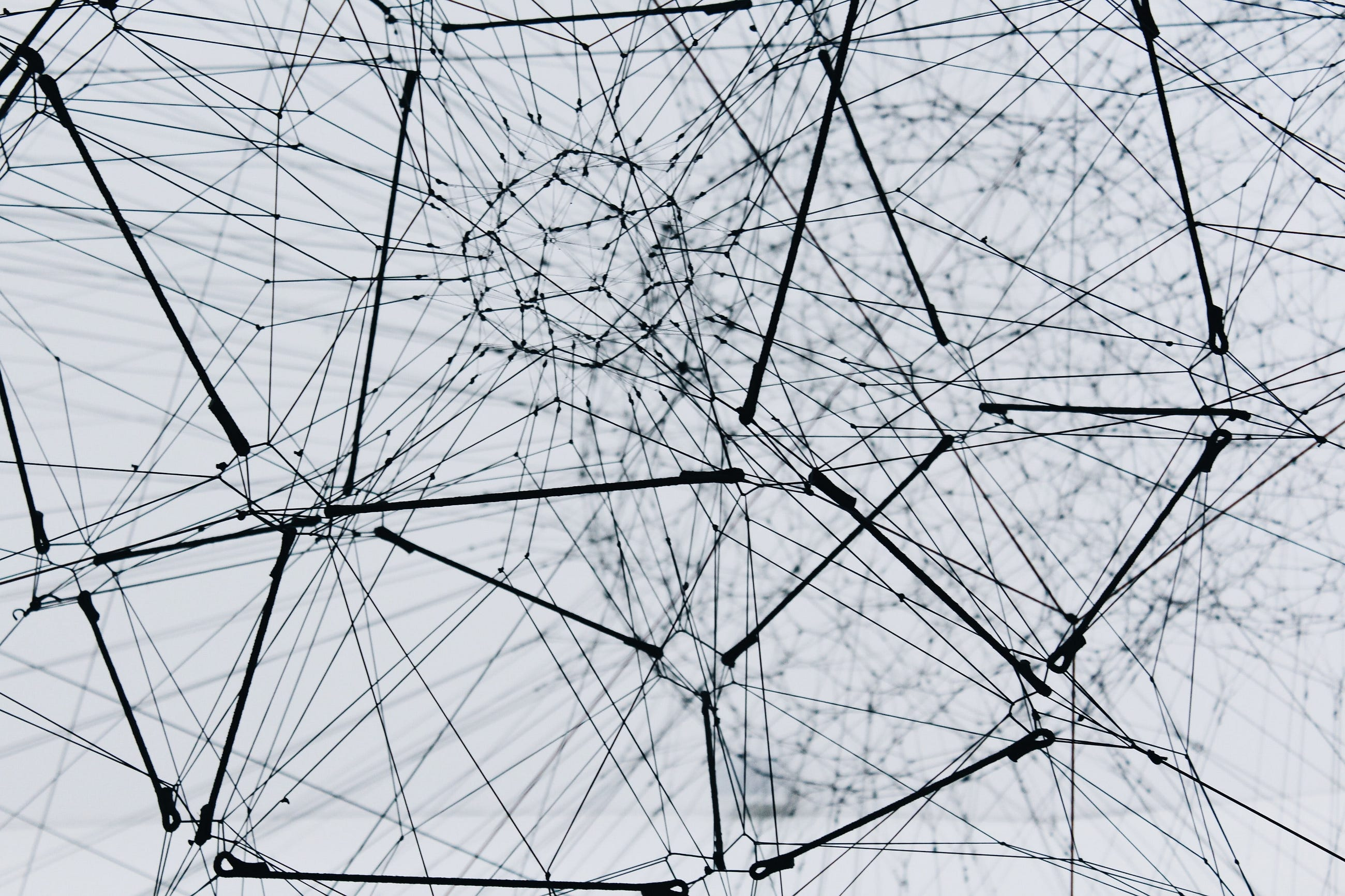# An Introduction to Graph Neural Networks (Part 1)

Original article was published by Arunkumar L on Deep Learning on Medium# An Introduction to Graph Neural Networks (Part 1)

## Graph Neural Networks and their applications

Over the past three years, Graph Neural Networks(GNN) has grown exponentially in popularity. This is due to their ability to learn from graph data efficiently. There is an intricate and cohesive network connection behind most systems that defines the interaction between the network components. This interaction can be represented as a graph. To model these systems, it is essential to understand the network behind them. Graph Neural Networks help us take advantage of the network’s relational structure to model it better and predict the outcome.

## What is a Graph?

A graph is a structured non-linear datatype with nodes(also called vertices) and edges. It is mathematically represented as G(V, E). For example, a molecule of NO₂ can be considered a graph where the nitrogen atom and two oxygen atoms are considered the node, and the bonds between the atoms are considered to be edges. Another example of a graph can be your family, where each person is a node, and the relationship between two people is the edge.

A graph can have labels on both its nodes and edges. It can either be numerical or textual. Each node will have some features that define the node. In a graph of NO₂, elements like the atomic number, atomic weight, and the number of valency electrons of each node can be its respective features. The edges might or might not have features depending on the type of graph. In NO₂, the edges’ features can be the bond strength, bond type(single bond or double bond), etc.

Graphs are classified on many different bases. The most common one is based on the edges of the graph. These are directed and undirected graphs. In a directed graph, the edges from one node to another are directional, while in an undirected graph, the nodes are connected through edges, and no direction is present.

A practical example of a directed graph is Instagram. When you follow someone, they don’t necessarily follow you back. In a sense, this is unidirectional. On the other hand, Facebook friend requests are an example of an undirected graph. Once the friend request is accepted, both of you can see each other’s content.

## Examples of Graphs

1. Social Networks: A social network is a graph where nodes represent people, and the relationship between two people is the edge. This relationship can be anything ranging from a simple acquaintance to a family.
2. Molecules: A molecule can be represented as a graph where the nodes represent the atoms, and the edges represent the bond between them.
3. Internet: The internet is a graph where the devices, routers, website, and the servers are the nodes and the internet connections are the edges.

## Analyzing Graphs (Elaborate)

1. Node Classification — Predict the type of a given node. For example, a given person in a social network can be classified based on their interests, beliefs, or characteristics.
2. Link Prediction — Predict if and how two nodes are linked. For example, finding if two given people(nodes) have any relationship between them.
3. Community Detection — Identify densely linked clusters of nodes. For example, finding if a group of people have any topic similarities.
4. Network Similarity — Measure the similarity of two nodes/networks. Here you can find if two people or two different groups of people are similar to one another.

## Some applications of Graphs Neural Networks

1. Recommendation systems: The abilities of a recommendation system can be increased exponentially using GNNs. With GNNs, the recommendations will be based on borrowing information from the nearby nodes, thus making the node embedding more accurate. Pinterest uses a GNN based recommender system.
2. Tackling misinformation: A hoax article can be identified using its links. Real articles link more coherently, while fake ones will have loose ends. According to a 2016 paper titled “Disinformation on the web,” humans can detect a hoax article 66 out of 100 times while a GNN can detect it 86 out of 100 times.
3. Drug development: All molecules can be represented as a graph. Using GNNs, it is possible to model complex networks like protein-protein interaction(PPI) networks and metabolic networks. This model helps in developing better and stable drugs for diseases.
4. Polarization on Twitter: Depending on the posts a person likes and people they follow, it is possible to detect if a person is polarized towards a specific view on a topic(political, environmental, etc.) or not.
5. Social Circle detection: Using GNNs, it is possible to detect a person’s social circle based on his interaction with others. This circle can be co-workers, college friends, family members, people in the same class, etc.

Why can convolutions not be applied to graphs?

Images have a fixed size and grid-based structure data with a defined spatial locality. On the other hand, graphs have arbitrary sizes, complex topology, and a non-euclidean structure. It also does not have a fixed node order. As we all know, neural networks are defined for specific sizes, grids, and structures. Thus convolutions cannot be directly applied to graphs.

## Conclusion

I’ll write about transforming the graphs into matrices like the Adjacency matrix, Incidence matrix, Degree matrix, and Laplacian matrix in the upcoming blogs. Further, I’ll discuss different approaches to using convolutions on graphs and their advantages and disadvantages, creating a node embedding of the graph, and forward propagation in GNN.